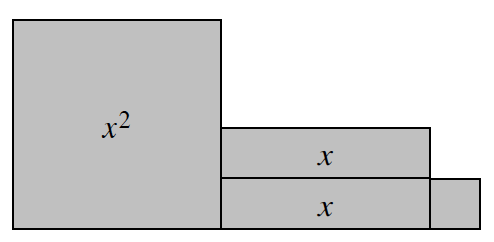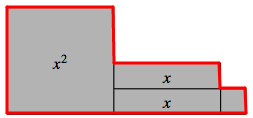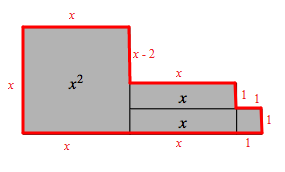### Home > ACC6 > Chapter 5 Unit 12 > Lesson CC2: 5.2.5 > Problem5-75

5-75.

Write expressions for the perimeter and the area of this algebra tile shape. Then simplify each expression by combining like terms.The perimeter is the distance around a shape. A bold line outlines the diagram.

Label the values of all of the sides. Added to the diagram, the Lengths of each side, starting in the upper left, going clockwise: x, (x minus 2), x, 1, 1, 1, 1, x, x, x.﻿

Add these values together to find the expression for the perimeter.
$x+x+(x-2)+x+1+1+1+1+x+x$
Simplify this expression by combining like terms.

$P=6x+2$

The area is the space bound within the perimeter.

Add the areas of all $4$ tiles within the perimeter.
$x^2+x+x+1$
Now simplify this expression.